# Weighted Finite State Transducers¶

Weighted Finite State Transducers are a powerful generalization of...

• hidden Markov models
• finite state machines
• regular expressions

Many algorithms that are quite a bit of work to implement "manually" can be expressed as simple operations on weighted finite state transducers, including:

• Viterbi
• forward-backward
• union, intersection, composition, closure, etc.

The OpenFST library is one of the most efficient and widely used libraries for weighted finite state transducers. It is being used with very large language models and works quite reliably.

All the examples given here are using Python bindings to the OpenFST library.

The original OpenFST library is in C++, and some of the C++ design and behavior is reflected in the Python bindings. Most importantly, many errors will simply terminate the OpenFST process, and with it the Python process (hopefully, better error handling hooks will be in the next release of OpenFST).

You can also use OpenFST directly from C++, and from the command line as well.

# Preliminaries¶

In :
from pylab import *
import openfst
from openfst import StdVectorFst as FST
from fstutils import *
figsize(12,4)


A simple function to read out a label sequence. openfst.GetString does something similar.

In :
def label_seq(fst,which=1,full=0):
result = []
total = 0
state = fst.Start()
while not fst.IsFinal(state):
assert fst.NumArcs(state)==1
a = fst.GetArc(state,0)
if which==0: l = a.ilabel
else: l = a.olabel
result.append(l)
total += a.weight.Value()
state = a.nextstate
result = [chr(c) for c in result if c>=32]
if full:
return result,total
else:
return "".join(result)


Before actually starting with the finite state transducers, let's first introduce the symbol table class. Internally, all inputs and outputs in the openfst library are integers, but for printing, that's not all that convenient. So, instead, we have a table that translates back and forth. For all these examples, we're just going to use ASCII characters. Symbol tables are stored with the transducers, allowing them to be interpreted even after saving.

In :
ASCII = openfst.SymbolTable("ASCII")
for i in range(127):
if i==0:
elif i<=32:
ASCII.AddSymbol("$%02x"%i,i) else: ASCII.AddSymbol(chr(i),i)  # Language Models¶ Although we are going to spend a lot of time talking about the details of finite state transducers, it is important to keep in mind that a finite state transducer is really just a large set of strings with associated costs. A simple language model can be implemented without all that machinery like, for example like this: In : class FiniteLanguageModel: def __init__(self,strings): self.strings = strings def cost(self,s): return self.strings.get(s,inf)  In : lm = FiniteLanguageModel({"hello":1.0,"world":1.5}) lm.cost("hello")  Out: 1.0 The second aspect of finite state transducers as language models is that they can be manipulated as objects in their own right. So, we can take two language models and combine them in some way. Here is a simple high level example: In : class UnionLanguageModel: def __init__(self,u,v): self.u = u self.v = v def cost(self,s): return min(self.u.cost(s),self.v.cost(s))  In : lm2 = FiniteLanguageModel({"the":0.5,"fox":0.7}) lm3 = UnionLanguageModel(lm,lm2) lm3.cost("fox")  Out: 0.7 Language models are a key part of speech recognition, handwriting recognition, and OCR. You can think of the recognition process as being divided into two parts: • the low-level recognizer analyzes sound or images and outputs a collection of recognition hypotheses with associated costs/probabilities • each recognition hypothesis is tested for its presence in the language model • the costs of the recognition hypothesis are combined with the costs from the language model • the result that has the lowest combined cost is returned by the system In principle, this seems straightforward, but the number of recognition hypotheses and the number of possible strings in the language model grows exponentially in the length of the input. This means that we need efficient algorithms for computing with these collections of strings. Weighted finite state transducers really give us a collection of capabilities: • they compact the set of strings in something like a finite language model into a small, efficient data structure • they allow statistically correct combinations of costs (where costs represent log proabilities) • they allow statistically correct combinations of multiple language models • they can represent infinite sets of strings • they can perform transductions: mappings from input strings to output strings with associated costs # Basic FST Construction¶ FST objects represent directed graphs in the form of a ragged array of nodes. In : fst = FST()  In : states = [fst.AddState() for i in range(3)]  In : def make_string_fst(s): fst = FST() states = [fst.AddState() for i in range(len(s)+1)] fst.SetStart(states) for i,c in enumerate(s): fst.AddArc(i,ord(c),ord(c),0.0,i+1) fst.SetFinal(len(s),0.0) return fst  In : fst1 = make_string_fst("hello")  In : arc = fst1.GetArc(0,0) arc.weight.Value()  Out: 0.0 The important parts of this data structure are: • The fst consists of a list of states; you need to add new states with AddState() • States are identified by integers; the total number of states is NumStates(). • Trying to use a non-existent state or arc will result in a segmentation fault (the underlying library is C++ w/o error checking) • Each state can be a start state (only one), and/or an accept state, optionally with an accept cost. For the arcs, you should know: • For each state, there is a list of arcs. • You can find the number of arcs with NumArcs(state) • You can get the i'th arc with GetArc(state,i) • Once you have an arc, it has properties ilabel, olabel, weight, and nextstate • The weight itself is an instance of a class. To get the numerical weight, use the Value() method on it. Let's illustrate this with a function that actually draws an FST. In : def show_fst(fst): import pydot,pylab graph = pydot.Dot(rankdir="LR") isyms = fst.InputSymbols() if not isyms: isyms = ASCII osyms = fst.OutputSymbols() if not osyms: osyms = ASCII for s in range(fst.NumStates()): if s==fst.Start(): n = pydot.Node("%d"%s,shape="box") graph.add_node(n) if fst.IsFinal(s): l = '"' l += "%d"%s # node id if fst.Final(s).Value()!=0.0: # optional non-zero accept cost l += "/%s"%fst.Final(s).Value() l += '"' n = pydot.Node("%d"%s,label=l,penwidth="3") graph.add_node(n) for t in range(fst.NumArcs(s)): a = fst.GetArc(s,t) l = '"' l += '%s'%isyms.Find(a.ilabel) if a.olabel!=a.ilabel: l += ":%s"%osyms.Find(a.olabel) v = a.weight.Value() if v!=0.0: l += "/%s"%v l += '"' n = a.nextstate e = pydot.Edge("%d"%s,"%d"%n,label=l) graph.add_edge(e) graph.write_png("/tmp/_test.png") pylab.gca().set_xticks([]); pylab.gca().set_yticks([]) pylab.clf() pylab.imshow(pylab.imread("/tmp/_test.png"))  In : show_fst(fst1)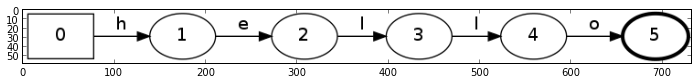In : def make_rstring_fst(s): fst = FST() states = [fst.AddState() for i in range(len(s)+1)] fst.SetStart(states) for i,c in enumerate(s): fst.AddArc(i,ord(c),ord(c),0.0,i+1) fst.AddArc(i,ord(c),ord(c),0.0,i) fst.SetFinal(len(s),0.0) return fst  In : fst2 = make_rstring_fst("hello") show_fst(fst2)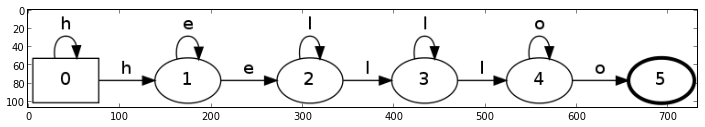# Built-In Constructors for Strings¶ There are some built-in constructors that make it easy to add strings to finite state transducers. In : fst = FST() fst.AddString("Hello") fst.AddString("World") show_fst(fst)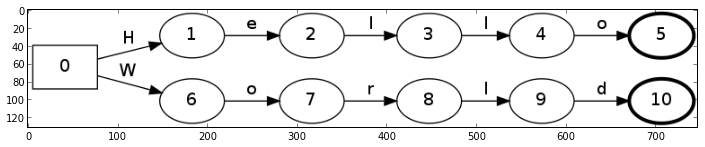In : fst = FST() fst.AddString("Hello",7.0,3.0,1.0) show_fst(fst)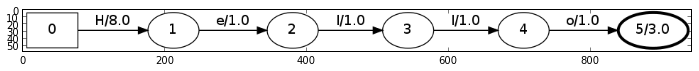In : fst = FST() fst.AddTranslation("Hello","World") show_fst(fst)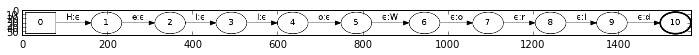# Union, Concatenation, ... and Epsilon¶ Finite state transducers are closely related to regular expressions, and there are a number of things we can do with regular expressions, such as concatenating them, making closures, etc. Several of these operations are built into openfst. In : figsize(12,4) a = FST() a.AddString("small") a.AddString("big") show_fst(a)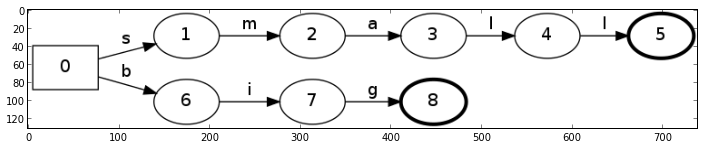In : b = FST() b.AddString("cat") show_fst(b)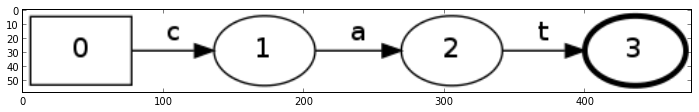In : openfst.ConcatOnto(a,b) show_fst(a)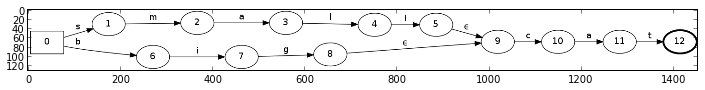Note the use of the epsilon symbol on arcs connecting the first and the second transducer. The epsilon symbol on a transducer consumes no input and produces no output. It just connects two states. In : a = FST() a.AddString("cat") show_fst(a)In : openfst.ClosureStar(a) show_fst(a)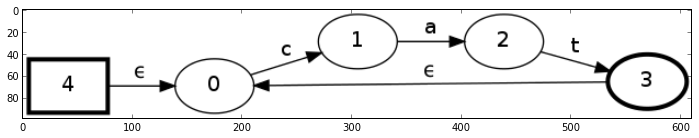In : a = FST() a.AddString("cat") openfst.ClosurePlus(a) show_fst(a)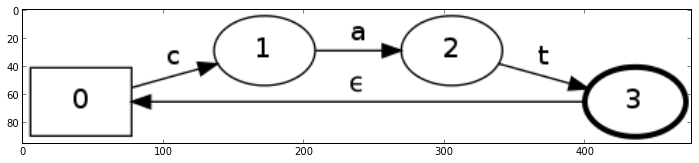In : a = FST() a.AddString("cat") b = FST() b.AddString("dog") openfst.Union(a,b) show_fst(a)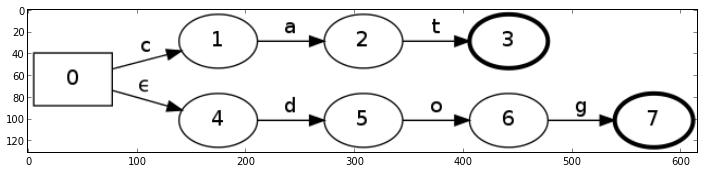# Weights¶ The weights in these computations are usually floating numbers, but with a pair of operations forming a "tropical semiring". In : w = openfst.Weight_Zero() print w.Value() w = openfst.Weight_One() print w.Value()  inf 0.0  The operations are those used for combining paths when they merge and for combining weights along a path. Common choices are (addition / multiplication / zero / one) • boolean: OR / AND / FALSE / TRUE (possibilities) • real:$+$/$*$/ 0 / 1 (probabilities) • log:$-\log(e^x+e^y)$/$+$/ inf / 0 (log probabilities, costs) • tropical:$\min$/$+\$ / inf / 0 (log probabilities, Viterbi)

Remember that these operations reduce to computations on conditional probabilities, either computing the maximum probability (minimum cost) to reach a node (Viterbi algorithm), or the total probability for reaching a node (forward algorithm).

# Infinite Sets of Strings and Sampling¶

Let's construct a transducer corresponding to "(cat|dog)+(ant|elk)+".

In :
a = FST()
openfst.ClosurePlus(a)
b = FST()
openfst.ClosurePlus(b)
openfst.ConcatOnto(a,b)
show_fst(a)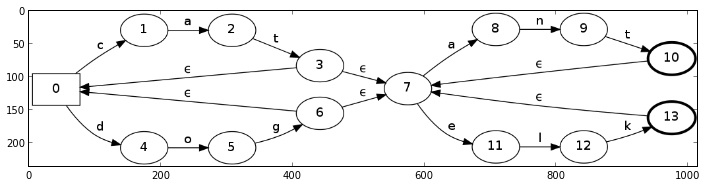As we said above, finite state transducers are collections of strings. We can pick a random sample from this collection with the RandGen function.

In :
out = FST()
openfst.RandGen(a,out)
print openfst.GetString(out)

catant


Written like that, the sampling algorithm makes a uniformly random choice at each node.

Since weights usually represent log probabilities, we may want to sample according to those. To do that, use RandGen and the LogProbArcSelector.

# Epsilon Removal¶

In :
a = FST()
b = FST()
openfst.Union(a,b)
show_fst(a)In :
openfst.RmEpsilon(a)
show_fst(a)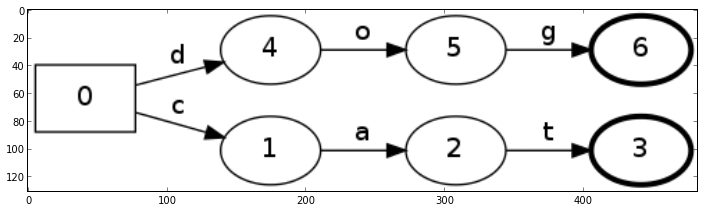In :
a = FST()
openfst.ClosureStar(a)
show_fst(a)In :
openfst.RmEpsilon(a)
show_fst(a)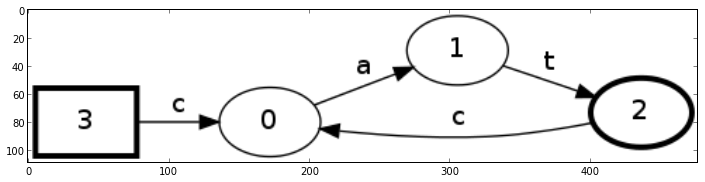Note that RmEpsilon removes transitions that have epsilon on both the input and the output. It gets more complicated when there are epsilons only on inputs or only on outputs.

In :
a = FST()
show_fst(a)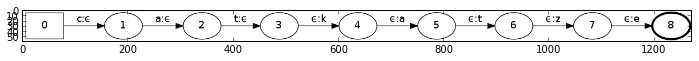In :
out = a.Copy()
openfst.RmEpsilon(out)
show_fst(out)In :
out = FST()
openfst.EpsNormOutput(a,out)
show_fst(out)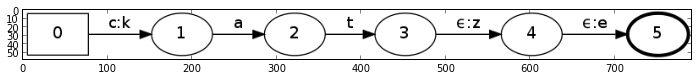# Determinization and Minimization¶

What makes finite state transducers so powerful is a number of operations that work generically on even very large and complex transducers.

Foremost among these are determinization and minimization.

## Unweighted, Acyclic¶

In :
fst = FST()
show_fst(fst)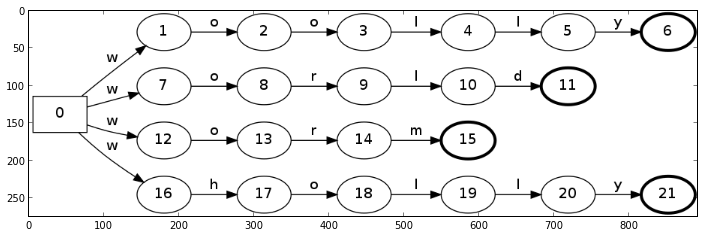The above machine is non-deterministic: starting in node 0, there are multiple arcs we can take, all labeled the same.

We can determinize this structure using openfst.Determinize. This yields a finite state transducer that is equivalent to a data structure called a trie.

In :
dfst = FST()
openfst.Determinize(fst,dfst)
show_fst(dfst)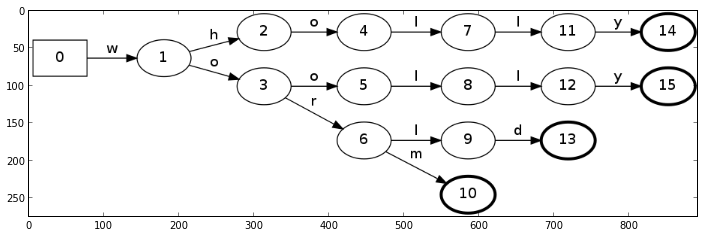We can compact this data structure further by minimization.

In :
openfst.Minimize(dfst)
show_fst(dfst)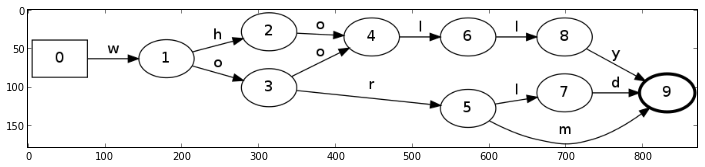In :
print fstsize(fst)
print fstsize(dfst)

(22, 21)
(10, 12)

In :
def minimize(fst):
dfst = FST()
openfst.Determinize(fst,dfst)
openfst.Minimize(dfst)
return dfst


Let's try to do this for something larger.

In :
fst = FST()
nlines = 0
with open("basic-english.txt") as stream:
line = line.strip()
nlines += 1
print nlines
print fstsize(fst)
fst = minimize(fst)
print fstsize(fst)

851
(4459, 4458)
(746, 1541)


## Weighted, Acyclic¶

In :
fst = FST()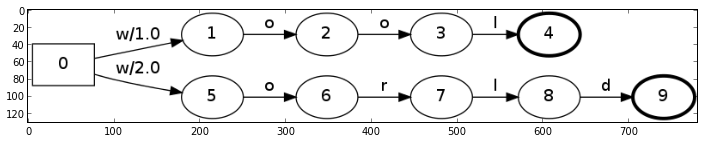dfst = FST()